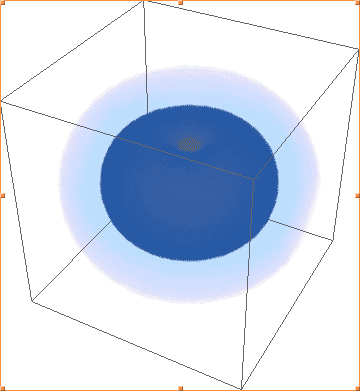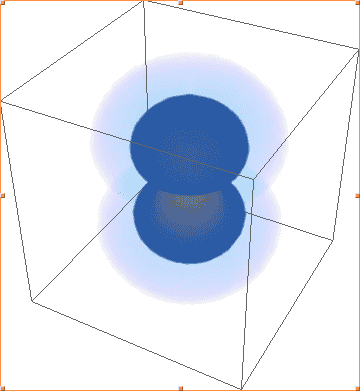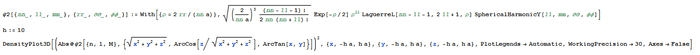# Plotting the Probability Density of the Coulomb Wave Function

Hey there - I think I have an issue with my 3D density plots of the probability density of the Coulomb wave function. The reason I think something is going wrong is because my plots of |ψ(n=2, l=1, m=-1)|² and |ψ(2, 1, 1)|² are identical, while I would expect them to have the same shape but be rotationally symmetric along different orthogonal axes.The above images are Mathematica's plots of |ψ(2, 1, -1)|², |ψ(2, 1, 0)|², |ψ(2, 1, 1)|², respectively. As you can see, the first and third are identical, and not the shape of 2p orbitals, while the second plot actually looks like what I would expect - one of the three 2p orbitals.

Here is my wave function - it's possible that the conversion from spherical to Cartesian coordinates is a problem, but I'm not sure:If the above needs clarifying, do ask. Thanks for any help in advance ;)

## Answers and Replies

fzero
Science Advisor
Homework Helper
Gold Member
jtbell
Mentor
If you take suitable linear combinations of ##\psi(2,1,-1)## and ##\psi(2,1,+1)##, and then find the probability densities, you do get the ##p_x## and ##p_y## orbitals.

If you take suitable linear combinations of ##\psi(2,1,-1)## and ##\psi(2,1,+1)##, and then find the probability densities, you do get the ##p_x## and ##p_y## orbitals.

Ah, I've managed to make linear combinations which give the x and y-direction p orbitals, but I am still confused - does that mean some orbital wave functions aren't energy energy eigenfunctions? How/why would that be true?

DrClaude
Mentor
Ah, I've managed to make linear combinations which give the x and y-direction p orbitals, but I am still confused - does that mean some orbital wave functions aren't energy energy eigenfunctions? How/why would that be true?
They are still energy eigenfunctions. However, ##p_x## and ##p_y## are not eigenfunctions of ##\hat{L}_z##.

They are still energy eigenfunctions. However, ##p_x## and ##p_y## are not eigenfunctions of ##\hat{L}_z##.

Right, I understand that, but I've now got more questions - what are those donut-shaped probability densities? Why aren't they valid orbitals? Also, is there a systematic way for me to combine these 'base' wave functions to create the correct electron orbitals? I managed to make an intuitive guess with the other two p orbitals, but is there a general set of linear combinations which give all of the orbitals?

Last edited:
DrClaude
Mentor
Right, I understand that, but I've now got more questions - what are those donut-shaped probability densities? Why aren't they valid orbitals?
They are completely valid orbitals. But they are complex functions, which can make them more difficult to work with in certain situations. The ##p_x## and ##p_y## orbitals are completely real functions, and their orientation along the Cartesian coordinates makes them useful to understand things like chemical bonding.

Also, is there a systematic way for me to combine these 'base' wave functions to create the correct electron orbitals? I managed to make an intuitive guess with the other two p orbitals, but is there a general set of linear combinations which give all of the orbitals?
See https://en.wikipedia.org/wiki/Atomic_orbital#Real_orbitals